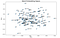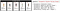# Text VectorizationVisualization of Word Embedding Vectors using Gensim and PCA (by Author)

# Build a KNN model

`indices = pd.Series(combined_df.product)#Build a KNN modelmodel = NearestNeighbors(n_neighbors=100,n_jobs=-1)knn = model.fit(combined_df_drop_product)def predict(name):dress_index = (combined_df[combined_df['product']==name]).index.tolist()    dress_index = dress_indexdistances, indices = knn.kneighbors(combined_df_drop_product.iloc[[dress_index],:])index = indices    distance = distancesnearest_list = []        for k in range(0,len(index)):        dress_name = combined_df.iloc[index[k],0]        if dress_name not in nearest_list:                nearest_list.append(dress_name)return nearest_list[1:]`Patagonia Alpine Valley Dress’s Recommended Products from the site V.S the Model Prediction (by Author)

# Product Reviews Sentiment Analysis

positive sentiment : (compound score >= 0.05)
neutral sentiment : (compound score > -0.05) and (compound score < 0.05)
negative sentiment : (compound score <= -0.05)

Written by